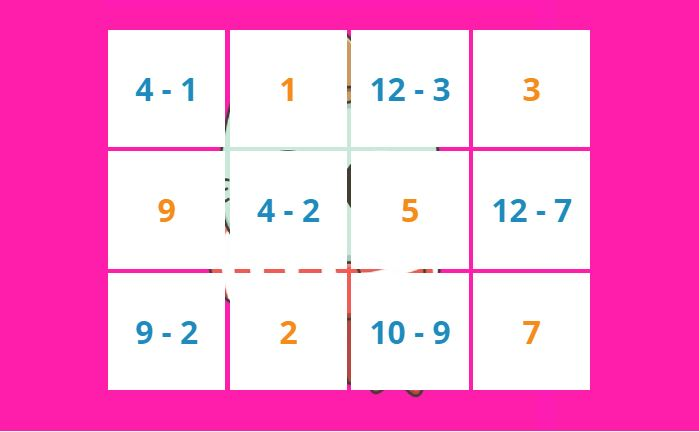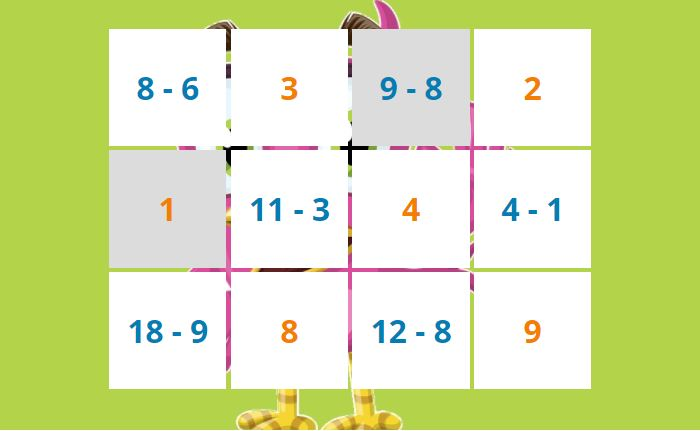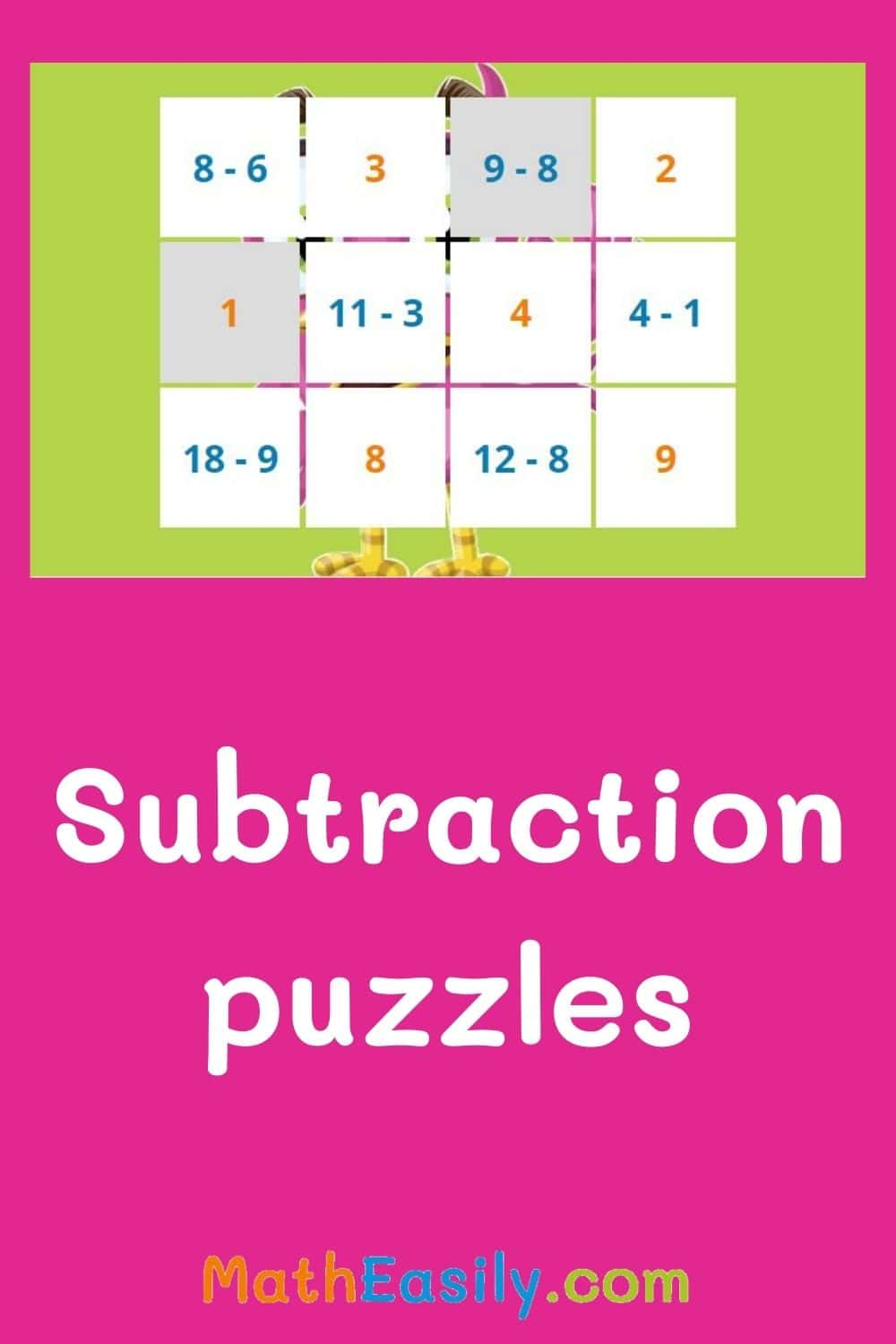﻿ Math subtraction puzzles up to 20

# Subtraction puzzles

Math subtraction puzzles for grade 1. addition and subtraction puzzles with answers. plus minus puzzle for kindergarten. addition subtraction puzzle up to 20. hidden picture subtraction. math puzzles addition and subtraction. maths addition and subtraction puzzles. math brain puzzles.

Click on 2 tiles so that they contain the same result. Watch as the hidden picture appears.

## How to play addition and subtraction puzzles?Half of the tiles contain the subtraction problem (blue text) and the other half contain the result (orange text).

Children have to click first on the task and then on the corresponding result. They are looking for pairs of subtraction puzzles: the task and the result.

When the kids manage to find a pair of tiles that belong together, the tiles disappear. When you click on 2 tiles which don´t match, the tiles will flash red.

Did you click on a tile by mistake? It doesn't matter, click it again, the tile will be white again.

Continue to play math subtraction puzzles until all tiles disappear. Then press the button "New puzzle" and a new subtraction puzzle appears.Playing hidden picture subtraction puzzles, children will easily learn to subtract up to 20. It also gives children a good foundation for learning to subtract larger numbers and to subtract without regrouping.

## Hidden picture subtraction puzzle worksheets

These online subtraction puzzles complement with hidden picture subtraction puzzle worksheets. Printable subtraction puzzles are designed for an use in the classroom or for home schooling. Everytime 12 new different subtraction problems within 20 are generated and create a new image. More than 50 pictures available.

Click the worksheet bellow and generate the new subtraction puzzle:

## Math subtraction puzzles for grade 1 or kindergarten

Hidden picture subtraction puzzles are a great method how children learn subtraction in a fun way. As kids complete the subtraction tasks, they gradually uncover cute pictures.

They look forward to what they see in the hidden picture and therefore find the subtraction problems easy.## Hidden picture subtraction puzzle up to 20

The addition and subtraction puzzle games take advantage of children's curiosity. Kids are excited to see what hidden picture will appear when they finish these math subtraction puzzles.

To practice subtraction browse our online subtraction games here or try these online subtraction flash cards, which are great for practicing and testing of subtraction skills.